## Data Structures Helpdesk

• Home
• Data Structures Helpdesk

## Online/Offline Data Structures Tutorials by Compuhelp Chandigarh

### Q. What is Data Structures?

A technique to store and orgainze the data efficiently in Computer.

### Q. What is Data ?

Data is defined as facts or figures, or information that's stored in or used by a computer

### Q. What is Information ?

The processed form of data is known as Information.

### Q. What are the Applications of Data Structures?

• Operating System.
• Artificial Intelligence.
• Computer Design.
• Computer Graphics.

### Q. What are the Types of Data Structures?

• Linear Data Structures
• Non-Linear Data Structures.

### Q. What are Linear Data Structures?

Linear data structures have data elements arranged in sequential manner and each element is connected to its previous and next element. Some Linear Data Structures are as follows Linear Data Structures.

• Arrays
• Stack
• Queue

### Q. What are Non-Linear Data Structures?

Data structures where data elements are not arranged sequentially or linearly. They are arranged in hierarchical form.Some Non-Linear Data Structures are as follows:

• Trees
• Graphs

### Q. What are the major Operations performed in Data Structures?

Insertion:
Insertion operation means addition of a new data element in a particular data structure.
Traversing:
Traversing operation means to visit each and every element of a particular Data Structure.
Deletion:
Deletion operation means removal of a given element from a particular Data Structure.
Searching:
Searching operation means to search a given element in a particular Data Structure.
Sorting:
Sorting operation means to arrange the elements in ascending or descending order in a particular Data Structure.

### Q. What is an Algorithm?

A sequence of steps or instructions to solve a particular problem. An algoritm has following properties:
Finiteness
The number of steps involved in an Algorithm to solve a problem must be finite.
Simplicity:
The steps involved in an algorithm must be simple, so that the output can be computed easily.
Input:
To solve any problem, the algorithm must recieve some input
Output:
The algorithm must produce at leat one output which is the solution to the problem.
Correctness
The algorithm must perform the task it is designed to perform, for all input values.
Generality
The procedure used in a specific algorithm should be applicable to all algorithms of same general form.

### Q. What is Time Complexity?

Time Complexity is the the measure of how long it takes for the algorithm to compute the required operation.

### Q. What is Space Complexity?

Space Complexity is generally used to refer to the measure of how much space an algorithm takes.

### Q. What is an Array ?

An array is a technique which is used to store more than one value but of similar type.It stores the values in contiguous or adjacent memory location.Every element of an array having its unique index number.
Instead of declaring individual variables, such as number0, number1, ..., and number99, we can declare one array variable such as numbers and use numbers, numbers, and ..., numbers to represent individual variables. A specific element in an array is accessed by an index.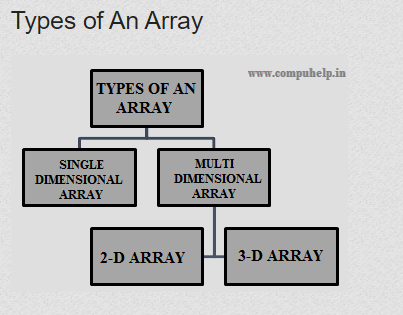### Q. What is Single Dimensional Array ?

An array of single or one dimension is known as a single dimensional array or 1-D array.
Single or one dimensional array can be of int,char,float etc.. type.### Q. What is Two Dimensional Array ?

An array of two dimension having rows and columns like of matrix is known as two dimensional array or 2-D array.
Two dimensional array can be of int,char,float etc.. type.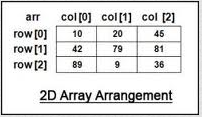### Q. What are the major operations Perfomed in Array?

The major operations performed in array are:
1. Insertion
2.Traversing
3.Deletion
4.Searching
5. Sorting

### Q. What is Insertion in an Array?

Insertion in an array here means insert an element in an array at a given location.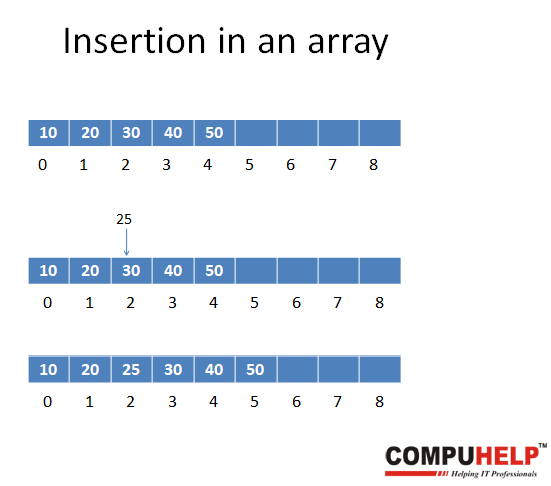### Q. Algorithm of Insertion in an Array.

INSERT(A,N,LOC,ITEM) [A is an array, N number of elements, LOC is location]
Step1. START
Step2. Repeat Step 3 For I<-N to I >= LOC, I<- I-1
Step3. A[I+1] <- A[I] [Moves elements with index I to Right by 1]
Step4. A[LOC] <- ITEM
Step5. N <- N+1 [Reset size to N+1]
Step6. END

### Q. Program of Insertion in an Array at a given location.

#include #include void insert(int array[],int *n,int item,int loc) { int i; for(i=*n-1;i>=loc;i--) { array[i+1]=array[i]; } array[loc] = item; *n=*n+1; }// end of insert function void main() { int array, loc, i, n, item; printf("Enter number of elements in the array\n"); scanf("%d", &n); printf("Enter %d elements\n", n); for (i = 0; i < n; i++) { scanf("%d", &array[i]); } printf("Please enter the location where you want to insert an item\n"); scanf("%d", &loc); printf("Please enter the item\n"); scanf("%d", &item); loc=loc-1; insert(array,&n,item,loc); printf("Resultant array is\n"); for (i = 0; i < n; i++) { printf("%d\n", array[i]); } getch(); }//end of main

### Q. What is Traversing in an Array?

Traversing in an array means to visit each and every element of a particular Data Structure and apply an operation on it.

### Q. Algorithm of Traversing in an Array.

Traversing in an Array.
Step1. START
Step2. ARR[MAX_SIZE], N number of elements, I for iteration
Step3. Repeat step 4 For I <- 1 to I <= N, I <- I + 1
Step4. Write(ARR[I])
Step5. END

### Q. Program of Traversing in an Array.

#include #include void traverse(int arr[],int n) { int i; for(i=0; i < n; i++) { printf("%d ",arr[i]); } } //end of traverse function void main() { int arr,n,i; printf("Enter number of elements in the array\n"); scanf("%d", &n); printf("Enter %d elements\n", n); for (i = 0; i < n; i++) { scanf("%d", &arr[i]); } printf("\n The traversed array elements are :"); traverse(arr,n); getch(); }//end of main function

### Q. What is Deletion in an Array?

Deletion in an array here means delete an element from a given location in an array.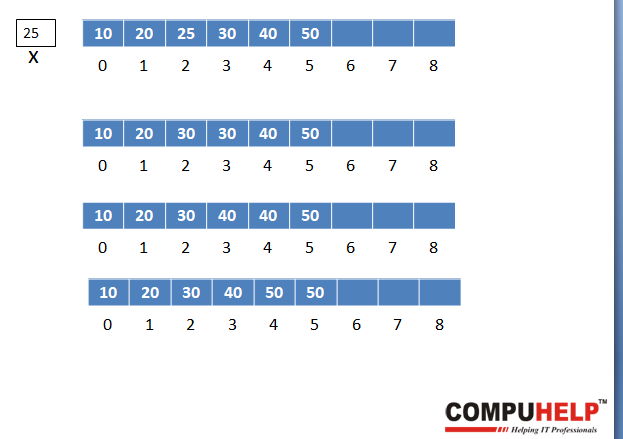### Q. Algorithm of Deletion in an Array?

Delete(A,N,LOC) [LOC is the location of the element to be deleted] Step1. START Step2. Repeat for I = LOC to N – 1, I<-I+1 A[I] <- A[I+1] [Move element with index I+1 left by 1] [End of loop] Step3. N <- N-1 [Reset the size of A to N-1] Step4. END

### Q. Program of Deletion in an Array.

#include #include void delete_array(int array[],int *n,int loc) { int i; if (loc >= *n+1 ) { printf("Deletion not possible.\n"); } else { for ( i = loc - 1 ; i < *n - 1 ; i++ ) { array[i] = array[i+1]; } *n=*n-1; } }//end of method delete_array void main() { int array, loc, i, n; printf("Enter number of elements in array\n"); scanf("%d", &n); printf("Enter %d elements\n", n); for ( i = 0 ; i < n ; i++ ) { scanf("%d", &array[i]); } printf("Enter the location from where you want to delete an element\n"); scanf("%d", &loc); loc=loc; delete_array(array,&n,loc); printf("Resultant array is\n"); for( i = 0 ; i < n ; i++ ) { printf("%d\n", array[i]); } getch(); }//end of main method

### Q. What is Searching in an Array?

Searching in an array means search the given element in the array and show the location.

### Q. What are the types of Searches in an Array?

There are two types of Searches in an array.
1. Linear Search.
2.Binary Search

### Q. What is Linear Search in an Array?

Linear search is a very simple search algorithm. In this type of search, a sequential search is made over all items one by one. Every item is checked and if a match is found then that particular item is returned, otherwise the search continues till the end of the elements in the array.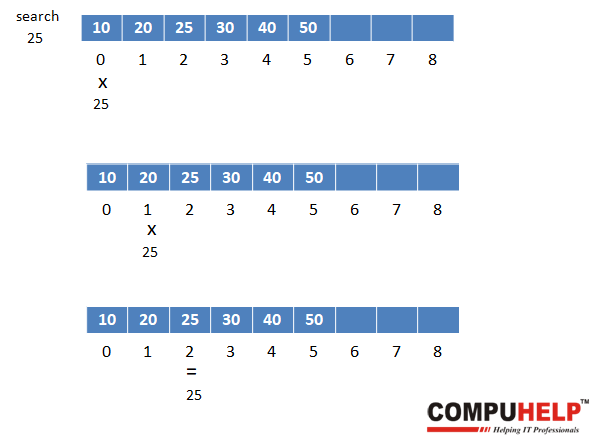### Q. Algorithm of Linear Search in an Array.

Linear Search(A,N,ITEM) Step1. START Step2. LOC <- -1 [Initialize Location Counter] Step3. I <- 1 [Initialize Counter] Step4. [Search for ITEM] Repeat while I N and A[I] ITEM I <- I + 1 [End of loop] Step5. If A[I] <- ITEM then [Successful] LOC <- I [End of If Structure] Step6. END

### Q. Program of Linear Search in an Array.

#include #include void linear_search(int arr[],int n,int element) { int i,flag=0; for(i=0;i

### Q. What is Binary Search in an Array?

Linear search is good for unsorted arrays. Linear search method is slow in large arrays. If array is of large size but sorted, we can use Binary search. It increases the speed of search operation.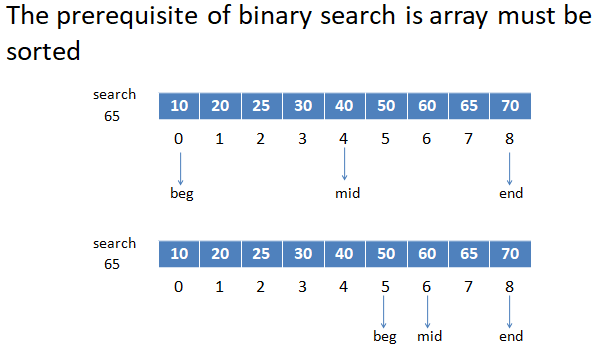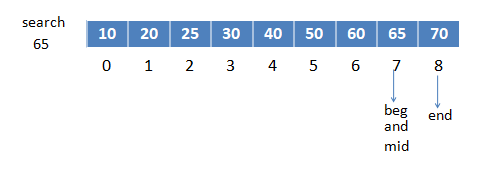### Q. Algorithm of Binary Search in an Array.

Step1. START Step2. LOC <- -1 [Initialize Location Counter] Step3. BEG <- 1, END <- N [Initialize] Step4. [Search for ITEM] Repeat steps 5 and 6 while BEG <= END[Traverse] Step5. MID <- (BEG+END)/2 Step6. If ITEM <- A[MID] then [Item found] LOC <- MID Exit loop Else If ITEM > A[MID] then [Look in second half] BEG <- MID + 1 Else [or Look in first half] END <- MID-1 [End of If Structure] [End of Step4 Loop] Step7. END Algorithm

### Q. Program of Binary Search in an Array.

#include #include int main() { int arr,i,item,n,loc,flag=0; int beg=0,end,mid; printf("Enter the number of elements :\n"); scanf("%d",&n); end=n-1; printf("Enter the array elements:\n"); for(i=0;i

### Q. What is sorting in an Array?

Sorting in an Array means arrange the elements of array in ascending or descending order.

### Q. What is Bubble Sort in an Array?

Bubble Sort is the simplest sorting that works by repeatedly swapping the adjacent elements of array if they are in wrong order.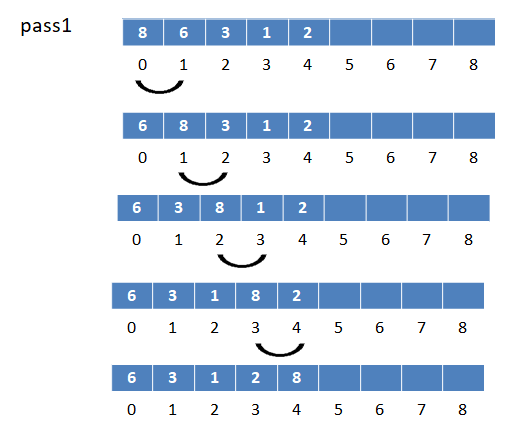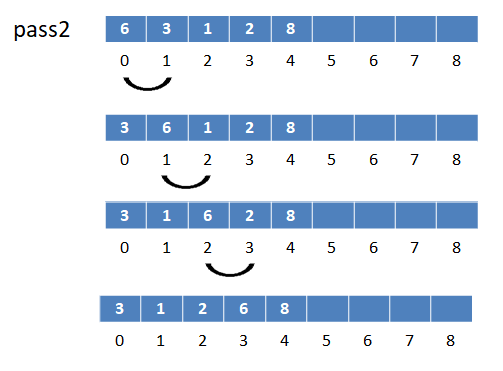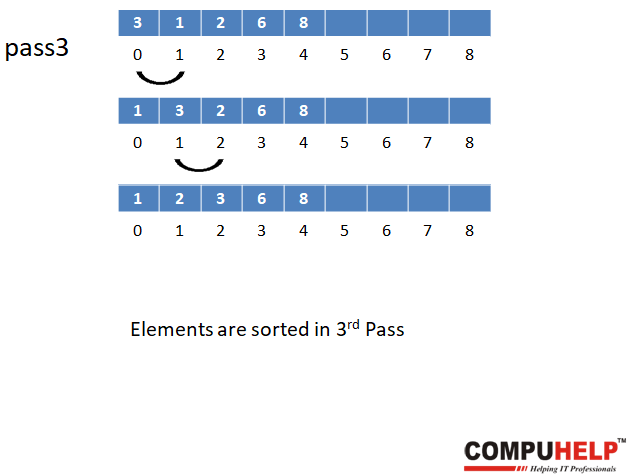### Q. Algorithm of Bubble Sort in an Array.

Bubblesort(A,N) – Given a one-dimensional array A with N elements. This algorithm sorts the array A according to the bubble sort technique. Step1. START Step2. Repeat Step 3 for I <- 1 to N-1[Number of Passes] Step3. Repeat Step 4 for J <- 1 to N-I Step4. If(A[J]>A[J+1]) then [Comparing adjacent elements] (a) TEMP <- A[J] (b) A[J] <- A[J+1] (c) A[J+1] <- TEMP [end of If Structure] [end of step 3 loop] [end of step 2 loop] Step5. END

### Q. Program of Bubble Sort in an Array.

#include #include void main() { int array, n, i, j, temp; printf("Enter number of elements\n"); scanf("%d", &n); printf("Enter %d Numbers:\n", n); for(i = 0; i < n; i++) { scanf("%d", &array[i]); } for(i = 0 ; i < n - 1; i++) { for(j = 0 ; j < n-i-1; j++) { if(array[j] > array[j+1]) { temp=array[j]; array[j]=array[j+1]; array[j+1]=temp; } } } printf("Sorted Array:\n\n"); for(i = 0; i < n; i++) { printf("%d\n", array[i]); } getch(); }//end of main function